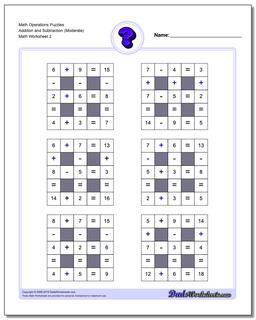PLEASE GO BACK AND USE THE BIG BLUE 'PRINT' BUTTON ON THE PAGE TO PRINT THE WORKSHEET CORRECTLY!Sorry for the trouble! The browser won't print the embedded worksheet PDF directly using the normal 'Print' command in the file menu, so you need to click the big 'Print' button to send just the worksheet and not the surrounding page to the printer.

# Math Worksheets: Number Grid Puzzles: Number Grid Puzzles: Math Operations Puzzles Addition and Subtraction (Moderate) (Second Worksheet)## Math Operations Puzzles Addition and Subtraction (Moderate) (Second Worksheet)

PropertyValue
DescriptionMath Operations Puzzles Addition and Subtraction (Moderate): Math puzzle worksheets that require students to fill in operations to complete the problems with numbers near 10. (Second Worksheet)
Resource TypeWorksheet